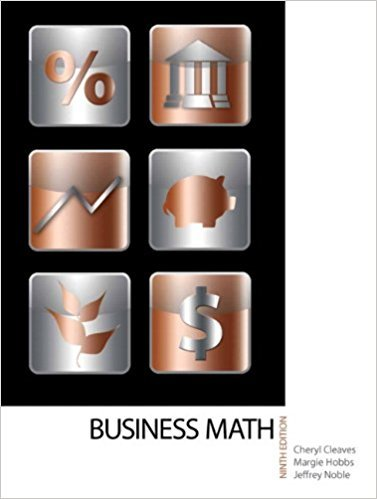×
×

# Solutions for Chapter 13-2: PRESENT VALUE## Full solutions for Business Math, | 9th Edition

ISBN: 9780135108178Solutions for Chapter 13-2: PRESENT VALUE

Solutions for Chapter 13-2
4 5 0 278 Reviews
26
1
##### ISBN: 9780135108178

Chapter 13-2: PRESENT VALUE includes 12 full step-by-step solutions. This expansive textbook survival guide covers the following chapters and their solutions. Business Math, was written by and is associated to the ISBN: 9780135108178. This textbook survival guide was created for the textbook: Business Math, , edition: 9. Since 12 problems in chapter 13-2: PRESENT VALUE have been answered, more than 17956 students have viewed full step-by-step solutions from this chapter.

Key Math Terms and definitions covered in this textbook
• Affine transformation

Tv = Av + Vo = linear transformation plus shift.

• Cholesky factorization

A = CTC = (L.J]))(L.J]))T for positive definite A.

• Companion matrix.

Put CI, ... ,Cn in row n and put n - 1 ones just above the main diagonal. Then det(A - AI) = ±(CI + c2A + C3A 2 + .•. + cnA n-l - An).

• Condition number

cond(A) = c(A) = IIAIlIIA-III = amaxlamin. In Ax = b, the relative change Ilox III Ilx II is less than cond(A) times the relative change Ilob III lib II· Condition numbers measure the sensitivity of the output to change in the input.

A sequence of steps (end of Chapter 9) to solve positive definite Ax = b by minimizing !x T Ax - x Tb over growing Krylov subspaces.

• Cramer's Rule for Ax = b.

B j has b replacing column j of A; x j = det B j I det A

• Diagonal matrix D.

dij = 0 if i #- j. Block-diagonal: zero outside square blocks Du.

• Dot product = Inner product x T y = XI Y 1 + ... + Xn Yn.

Complex dot product is x T Y . Perpendicular vectors have x T y = O. (AB)ij = (row i of A)T(column j of B).

• Factorization

A = L U. If elimination takes A to U without row exchanges, then the lower triangular L with multipliers eij (and eii = 1) brings U back to A.

• Fibonacci numbers

0,1,1,2,3,5, ... satisfy Fn = Fn-l + Fn- 2 = (A7 -A~)I()q -A2). Growth rate Al = (1 + .J5) 12 is the largest eigenvalue of the Fibonacci matrix [ } A].

• Fourier matrix F.

Entries Fjk = e21Cijk/n give orthogonal columns FT F = nI. Then y = Fe is the (inverse) Discrete Fourier Transform Y j = L cke21Cijk/n.

• Gram-Schmidt orthogonalization A = QR.

Independent columns in A, orthonormal columns in Q. Each column q j of Q is a combination of the first j columns of A (and conversely, so R is upper triangular). Convention: diag(R) > o.

• Kirchhoff's Laws.

Current Law: net current (in minus out) is zero at each node. Voltage Law: Potential differences (voltage drops) add to zero around any closed loop.

• lA-II = l/lAI and IATI = IAI.

The big formula for det(A) has a sum of n! terms, the cofactor formula uses determinants of size n - 1, volume of box = I det( A) I.

• Linearly dependent VI, ... , Vn.

A combination other than all Ci = 0 gives L Ci Vi = O.

• Orthogonal subspaces.

Every v in V is orthogonal to every w in W.

• Schur complement S, D - C A -} B.

Appears in block elimination on [~ g ].

• Skew-symmetric matrix K.

The transpose is -K, since Kij = -Kji. Eigenvalues are pure imaginary, eigenvectors are orthogonal, eKt is an orthogonal matrix.

• Solvable system Ax = b.

The right side b is in the column space of A.

• Spectral Theorem A = QAQT.

Real symmetric A has real A'S and orthonormal q's.

×